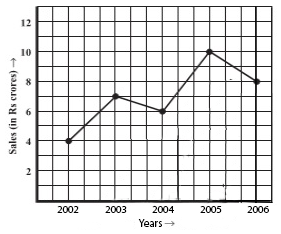# Introduction to Graphs

This is an MCQ-based quiz on Introduction to Graphs.

This includes Bar-graph, Pie-chart, Histograph, and Line Graph

Start Quiz

A ________ is a bar graph that shows data in intervals.

Bar-graph Pie-chart Histograph Line Graph

A graph that displays data that changes continuously over periods of time is called:

Bar-graph Pie-chart Histograph Line Graph

A line graph which is a whole unbroken line is called a:

Linear graph Pie-chart Histograph Bar-graph

Which point lies of y-axis?

(-2, 0) (2, 0) (0, -2) (2, -2)

If we join (4,2), (4,-3) and (4,4), then we obtain:

A triangle Straight-line without passing through origin Straight-line passing through origin None of the above

The point (9,0) lies on which of the following?

X-axis

Y-axis

Origin

None of the above

The point (4,-4) is:

Near to x-axis

Near to y-axis

Near to origin

Equidistant from x-axis and y-axis.

The point (10, 2) is nearer to:

X-axis

Y-axis

Origin

None of the above

Which of the following statements is/are true?

The X-axis is a vertical line. The Y-axis is a horizontal line. The scale on both the axes must be the same in a Cartesian plane. The point of intersection between the X-axis and Y-axis is called the origin.

In which quadrant does the point Q (-2, -6) lie?

I II IV III

Which of the points given is a point on the X-axis?

(5,0) (0,5) (5,3) (3,5)

On which axis does the point (-1, 0) lie?

X-axis

Origin

Y-axis

None of these

The point (-2, -5) is nearer to:

x-axis

y-axis

Origin

None of the above

The line graph shows the yearly sales figure for a manufacturing company. From the graph, what were the sales in 2006?Rs 8 crore

Rs 4 crore

Rs 3 crore

Rs 5 crore

Quiz/Test Summary
Title: Introduction to Graphs
Questions: 14
Contributed by: